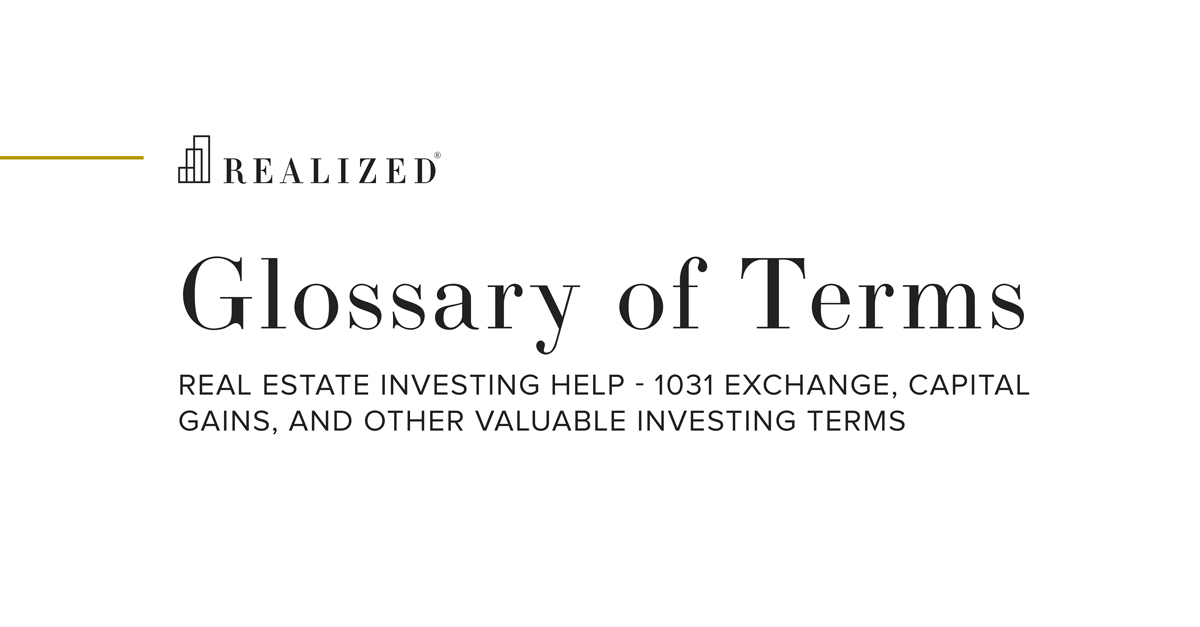Sharpe Ratio 2020-07-06 08:00:00

The sharpe ratio is a measure of investment return against risk. The higher this ratio, the less risk one is taking for return. The sharpe ratio formula is (return - risk free rate) / [standard deviation]. We want to know the risk-adjusted return, which the following gives us: return - risk free rate. Volatility, or risk, is found from the standard deviation. The larger our return (numerator) and the smaller the risk (denominator), the larger our sharpe ratio will be. As risk increases without an equal or greater increase in return, the sharpe ratio will drop, signifying we are taking on more risk for each unit of return. When comparing sharpe ratios across investments, it’s important to compare similar investments.

### Manage risk and help maximize opportunity